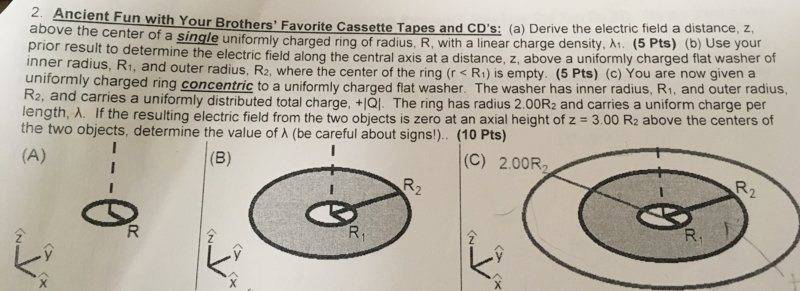# Electric field of ring of charge/washer of charge/disk of charge

In summary, Homework Equations:-charge density equations-electric field equations-surface charge-integral form of electric field-solution for electric field in a cutoutf

## Homework Statement## Homework Equations

charge density equations, electric field equations,

## The Attempt at a Solution

My attempts are attached. The attachment labeled A, is part A. Part B took a lot of paper so there are two attachments labeled B1 and B2. It is really B and C that I am struggling with. Not sure if I am on the right track or if it is correct. Any help would be much appreciated!

A little more thought: All the problems I have done in the back of my physics textbook are not like part B. I can find the electric field of a disk without a cut out in it. My thought process of finding the electric field with a cut out in it is integrating from the smaller radius to the larger radius. However, The total area of the washer is piR2^2-piR1^2. I plugged this result into my surface charge density toward the end of my solution.

My attempt at solution C:
Washer has charge +Q, Ring has radius 2R2, Lambda=Q/L
Clearly the charge on the ring has to be negative
therefore Lambda on ring is: -Q/4piR2

#### Attachments

Last edited:
B1.JPG is correct. APG is also correct. Combine the two results and set it equal to zero with ## z=3 R_2 ##. ## \\ ## In APG, you must set ## Q=2 \pi (2R_2) \lambda=4 \pi R_2 \lambda ##, and ## R=2R_2 ##. (This is not the same ## Q ## that you have in B1.JPG. so call it ## Q' ##. It turns out ## Q' \neq Q ##. ). ## \\ ## Suggestion: Call the ## E_z ## at ## z=3R_2 ## by ## E_1 ## and ## E_2 ##. You must have ## E_1+E_2=0 ##, which means ## E_2=-E_1 ##. Your answer above for ## \lambda ## is incorrect, but the rest is really just algebra. Try again. I'll be happy to check your result. (Note: ## E_2 ## is an expression that has ## \lambda ## in it, and ## E_1 ## has ## Q ## in it. Using ## E_2=-E_1 ##, solve for ## \lambda ##).

Last edited:
•Thank you! I’ll work on it tomorrow and respond! Appreciate it

•B1.JPG is correct. APG is also correct. Combine the two results and set it equal to zero with ## z=3 R_2 ##. ## \\ ## In APG, you must set ## Q=2 \pi (2R_2) \lambda=4 \pi R_2 \lambda ##, and ## R=2R_2 ##. (This is not the same ## Q ## that you have in B1.JPG. so call it ## Q' ##. It turns out ## Q' \neq Q ##. ). ## \\ ## Suggestion: Call the ## E_z ## at ## z=3R_2 ## by ## E_1 ## and ## E_2 ##. You must have ## E_1+E_2=0 ##, which means ## E_2=-E_1 ##. Your answer above for ## \lambda ## is incorrect, but the rest is really just algebra. Try again. I'll be happy to check your result. (Note: ## E_2 ## is an expression that has ## \lambda ## in it, and ## E_1 ## has ## Q ## in it. Using ## E_2=-E_1 ##, solve for ## \lambda ##).
Okay, So I am finally looking at your response in more detail.
What I have so far is:
E2 is electric field for concentric ring
E2=(k)(lambda)(dl)/r^2
I see what you mean that E1 + E2 has to = 0. Therefore E2=-E1. I guess my question is did I already solve for E1 in image B1JPG?

Yes, that is ##E_1 ## and it is correct.## \\ ## And ## E_2 ## is correct in A1JPG, but you need to set ## R=2R_2 ##, and ## Q =2 \pi (2R_2) \lambda ##. (As previously mentioned, this is not the same ## Q ## that it in B2jpg).## \\ ## In your post 4 here, ## E_2 ## is missing the ## \cos(\theta) =\frac{z}{(R^2+z^2)^{1/2} } ## factor.

Last edited:
Okay, I believe I see what you are saying-
Ering= (kλ2pi2R2z)/(2R22 + z2)(3/2)
and
EDisk= (2pizQ)/((4piε0(piR22-piR12)) [1/(z2+R12)1/2-1/(z2+R22)1/2]

Then I say ERing+EDisk=0 and solve for lambda?
That seems like some really tedious algebra which I haven't tried yet. Wanted to know if this was the correct method before I try to do it.
Thanks!

Okay, I believe I see what you are saying-
Ering= (kλ2pi2R2z)/(2R22 + z2)(3/2)
and
EDisk= (2pizQ)/((4piε0(piR22-piR12)) [1/(z2+R12)1/2-1/(z2+R22)1/2]

Then I say ERing+EDisk=0 and solve for lambda?
That seems like some really tedious algebra which I haven't tried yet. Wanted to know if this was the correct method before I try to do it.
Thanks!
Quite a bit of that "cancels out" . You should either use Coulomb's coefficient, ##\ k\ ##, for both or ##\ \displaystyle \frac{1}{4\pi \varepsilon_0}\ ##. I suggest the latter. Gets rid of some ##\ \pi \, ##s .

You have: ##\ \displaystyle E_{Ring} = \frac{kλ2\pi 2 R_2\, z} { \left( 2{R_2}^2 + z^2 \right)^{3/2}}\ ## but I suppose you should be squaring (2R2), not just the R2 .

Changing to ε0 form and the above correction gives:
##\ \displaystyle E_{Ring} = \frac{2\, \lambda \pi \, 2 R_2\, z} { 4\pi\,\varepsilon_0 \left( {(2R_2)}^2 + z^2 \right)^{3/2}}\ ##​

The expression for the disk is:
##\ \displaystyle E_{Disk} = \frac{2\pi z \,Q} {(4 \pi \varepsilon_0) \left(\pi {R_2}^2 - \pi {R_1}^2 \right)}
\left(\frac{1}{( z^2 + {R_1}^2 )^{1/2} } - \frac{1}{(z^2 + {R_2}^2 )^{1/2} } \right) ##
.

Last edited:
•Okay! I’ll try it out! Thanks

Okay! I’ll try it out! Thanks
I was editing that for a while. Just got finished.

•